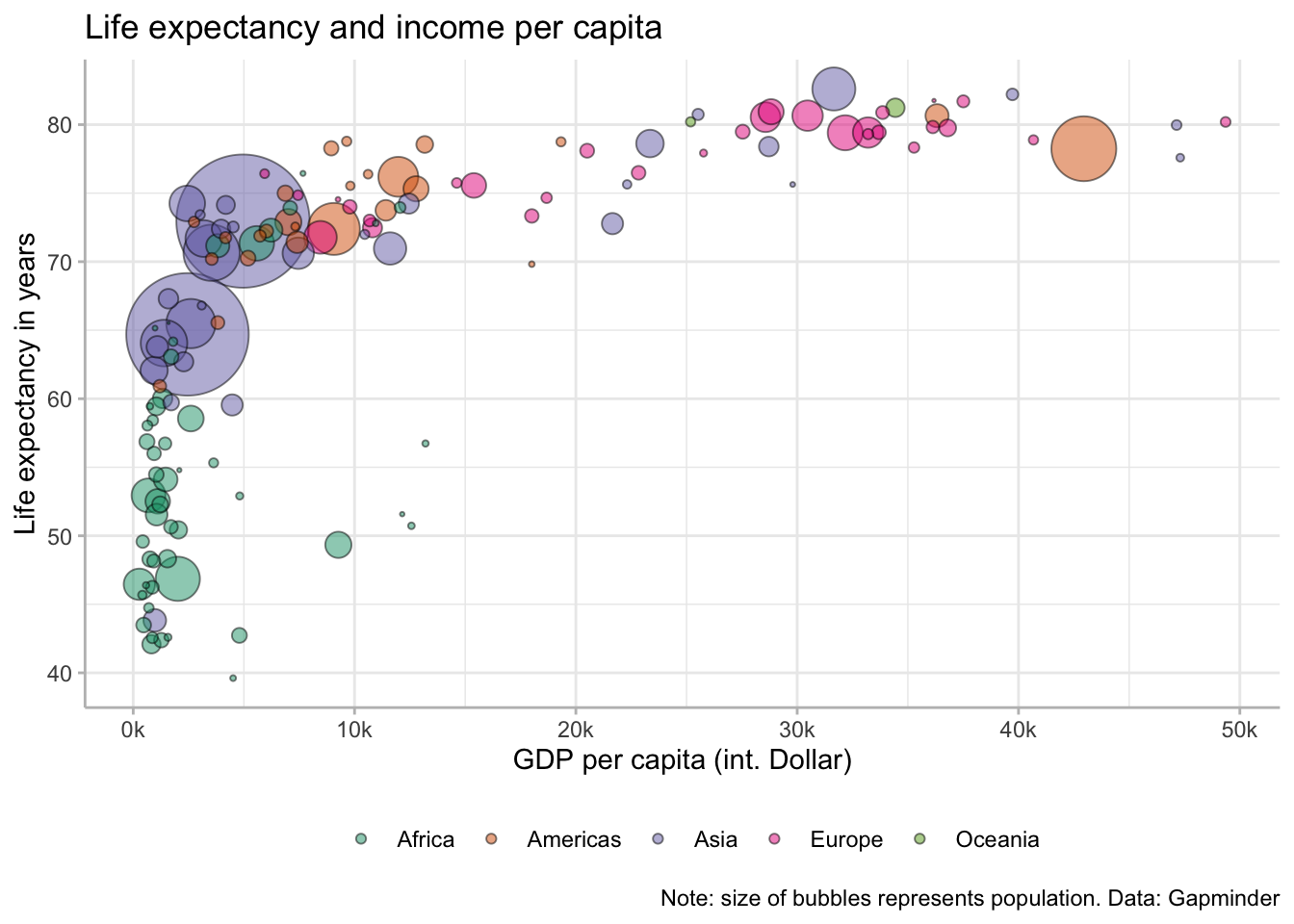# 1 Packages used

``````library(DataScienceExercises)
library(ggplot2)``````

# 2 Developing a ggplot - the general workflow

Make a shortcut to the data and inspect it:

``````gdp_data <- DataScienceExercises::gdplifexp2007
``````##         country continent lifeExp        pop gdpPercap
## 1         China      Asia  72.961 1318683096  4959.115
## 2         India      Asia  64.698 1110396331  2452.210
## 3 United States  Americas  78.242  301139947 42951.653``````

Plots in `ggplot2` are created layer by layer. We now go through each step that, in the end, will produce the following plot:We start by creating the basic `ggplot2` object, which is best thought of as a fancy list. To this end we use the function `ggplot2::ggplot()`

``````gdp_plot <- ggplot2::ggplot()
typeof(gdp_plot)``````
``##  "list"``

When we call this list, the plot described by it gets rendered:

``gdp_plot``Of, course, there is no plot since the list is basically empty. All the specifications in the `ggplot2::ggplot()` function are best thought of as default values. In our case we fist specify the data set we use for our plot:

``````gdp_plot <- ggplot2::ggplot(
data = gdp_data
)``````

But this alone does not do anything good. We also need to inform `ggplot2` on how it should map the variables from the data set onto the plot. In a first step, lets clarify that the variable `gdpPercap` should be mapped on the x-axis and the variable `lifeExp` on the y-axis.

This is done via the argument `mapping` and the function `ggplot2::aes()`, which takes as arguments the aesthetics of the plot and the variable names that should be plotted on them:

``````gdp_plot <- ggplot2::ggplot(
data = gdp_data,
mapping = ggplot2::aes(
x = gdpPercap,
y = lifeExp
)
)
gdp_plot``````